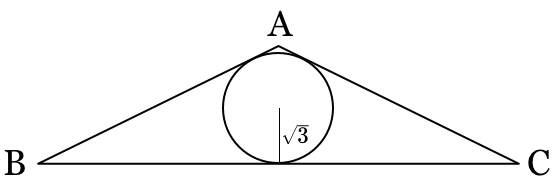# Area of a Triangle Just From a Circle?The given triangle is isosceles with vertex angle $120^\circ$ and inradius of length $\sqrt{3}.$ In simplest form, the area of the triangle is $a + b\sqrt{c}.$ Find $a+b+c.$

×